### Home > INT3 > Chapter 5 > Lesson 5.2.3 > Problem5-81

5-81.
1. This problem is a checkpoint for transformations of functions. It will be referred to as Checkpoint 5.

2.Make a complete graph of each function below without using a graphing calculator. State the family of functions, and describe how each coefficient in the equation transforms the location or shape of the graph in relation to the parent function. Homework Help ✎

3.  a. f(x) =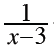+ 4 b. h(x) = 3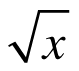Write an equation in graphing form for each graph below. c.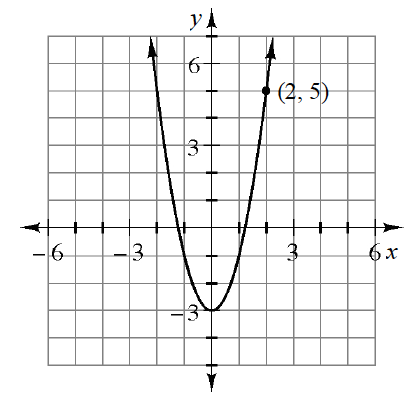d.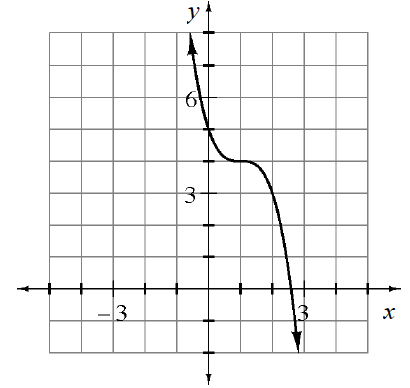4. Check your answers by referring to the Checkpoint 5 materials located at the back of your book.

Ideally, at this point you are comfortable working with these types of problems and can solve them correctly. If you feel that you need more confidence when solving these types of problems, then review the Checkpoint 5 materials and try the practice problems provided. From this point on, you will be expected to do problems like these correctly and with confidence.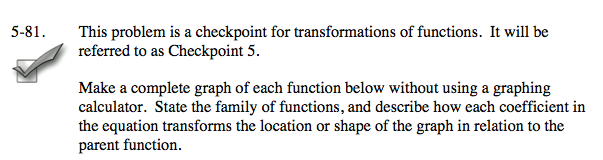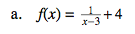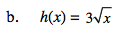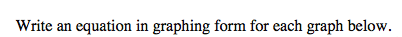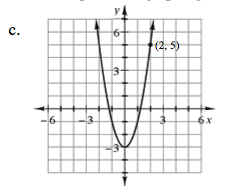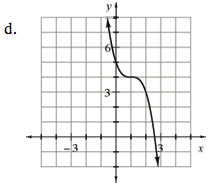Answers and extra practice are located in the back of your printed textbook or in the Reference tab of your eBook.
If you have an eBook, login and then click the following link: Checkpoint 5 Answers and Practice